# C Functions

The C functions are another important feature in C language which helps in achieving a particular task. There is at least one function in a C program and it’s called the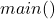function. Without the main, there is no C program.

You cannot delete the main function, but you can delete other user-defined functions.

## Why Functions ?

There are two reasons why function exists

1. Code reuse
2. Better program management because programming task can be broken and distributed to many functions.

You can repeat the same function by calling instead of writing the whole code again as many times as required in your C programs.

In structured programming practice, the program is broken into manageable tasks and later each task becomes a function with own codes. This is very easy to manage compared to writing whole code into thefunction itself.

## Important parts of a C function

Three things are important about a C function

1. Function declaration
2. Function definition
3. Function call

### Function Declaration

You can declare function prototype using formal argument in two location in a C program.

• Global declaration
• Local declaration

Some declaration is done outside offunction and those are called the globally declared variables or functions.

There is nothing special about them except that you can call them anywhere in your program or another file in the same program.

The scope of a local variable is inside of main or inside of a user-defined function. It means you cannot call the function outside oror another user-defined function.

Syntax: –

data_type function_name (
data_type variable1,
data_type variable2,
.
.
data_type variable n);

Example: –

int addition (int a, int b);

### Function definition

The function definition is written outside of thefunction and it has all the statements to perform a specific task assigned to that function. You can declare local variables other than function parameters in a function definition.

Syntax:

data_type> function_name (data_type variable1…)
{
Statement 1;
Statement 2;
.
.
Statement n;
}

Example:

int addition (int x, int y)
{
int result = x + y;
return result;
}

### Function Call

You can call a function anywhere you want to use it depending on the scope of the function. You can call a function in three places

• Inside another function
• Inprogram
• Another file in the same program

Syntax:

function_name (variable_list);

Example:

addition (a, b);

## Function Types

Earlier you learned about the function components inside a C program. There are different types of functions in C language. They are as follows

1. Function without parameters
2. Function with parameters
3. Function with return
4. Function without return

We will discuss each one of them in more details.

### 1. Functions without parameters

These type of functions do not take parameters to perform a computation.

Syntax:

data type function_name> ( );

Example:

int addition ( );

### Example Program:

#include <stdio.h>
int main ()
{
int result = 0;
int addition (); /* function declaration */
result = addition (); /* function call */
printf ("Total= %d\n", result);
getch ();
return 0;
}
/* function definition */
{
int a = 10;
int b = 20;
int total = 0;
total = a + b;
return output;
}

### Output

Total = 30

### 2. Function with parameters

A function with parameter has two important parameter values depending on where it appears.

1. Formal parameters
2. Actual parameters

Formal Parameters

The formal parameter appears at the function declaration when you tell the kind of argument is used, especially the data type.

For example

void sum (int x, int y);

and

void sum (int, int);

They are the same type of declarations.

Actual Parameters

The actual parameters are only used in function calls. The things you must know about functions with parameters are

What kind of values they take?

1. Integer
2. Float or double
3. Array
4. String of characters
5. Pointers

Syntax:

data_type function_name ( variable1 …);

Example:

void multiply (int a, int b);

How to supply parameters for these types of functions during function call?

There is two way to do this

1. Call-by-Value
2. Call-by-Reference

If you want to learn the how this works – check examples of both

## Examples of each input

In this section, we will discuss different calls to functions.

### Integer value

Example:

int a, b;
a = b = 10;
/* function declaration */
int addition (int a, int b);
/* function call */
/* function definition */
int addition (int a, int b)
{
do something;
}

### Float or Double

Example:

float a, b;
a = b = 10.45;
/* function declaration */
float addition (int a, int b);
/* function call */
/* function definition */
float addition (float a, float b)
{
do something;
}

### Character value

Example:

char vote;
vote = 'Y';
/* function declaration */
void opinion (char vote);
/* function call */
opinion (vote);
/* function definition */
void opinion (char vote)
{
do something;
}

### Array value as parameter

An array is a contagious memory location that holds the same type of values, and a subscript helps in identifying the correct location of an array element. There are four notations to access the value from an array.

1.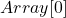– refers to value of first element of array.
2.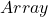– refers to memory address of first element of an array.
3.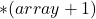or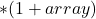– refers to value of second element of an array.
4.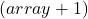or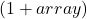– refers to memory address of second element of an array.

Note: The notation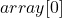is same as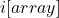because it meansor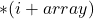which called the commutative property.

When you use an array as a parameter to function.

• The name of the array ‘array’ is a pointer to the first location in an array. It contains the memory address of the first element in the array.
• The index or subscript is added to the memory address to find all elements of the array which is much more efficient than copying and passing an entire array and slowing the program.

Since it is not possible to send the entire array as a function argument by call-by-value method, passing a pointer to the first element or passing the address to the first element is easy. Later any element of the array can be accessed using subscript or index value.

Syntax:-

/* Function declaration */
int sum (int arr []);
/*Function call where arr points to the first element of the arr [] */
sum (arr)
/* Function definition */
int sum (int arr []) {
do something;
}

### Pointer value as parameter to function

Pointers do not hold any value, but points to the memory address of a variable that has a value.

There are some rules regarding the pointer.

1. Pointer contains memory address of variable
2. Pointer must point to something before you use them.
3. Pointer is passed as parameter to a function using call-by-reference method.

Here is an example of pointer as function parameter.

Syntax:

/* Function declaration */
int sum (int *p);
int a = 10;
p = &a;
/*Function call where 'p' points memory address of variable 'a' */
sum (p);
/* Definition of the function*/
int sum (int *p);
{
Do something;
}

### 3. Function with return

After completing a specific task some function return a single value to the main function or to a function that called it.

A function with return requires two things.

1. A return statement using keyword –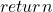.
2. The return type should match with the return type of function.

Syntax:

data_type function_name ( )
{
do something;
return variable;
}

Example:-

int sum (int a, int b)
{
int sum = 0;
sum = a + b;
return sum;
}

Notice that the sum is an integer and returns an integer value which matches with the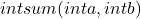function data type. If there is a mismatch you will get an error.

The main function always returns an integer by default, unless it’s specified not to return anything.

### 4. Function without return

There is a different class of functions that do not return any value. There are two requirements to define such a function.

1. No return keyword required because function does not return any value.
2. The function has no data type declared and instead the keyword ‘void’ is used to denote that the function does not return anything.
void sum (int a, int b)
{
int sum = 0;
sum = a + b;
printf ("The sum is %d\n", sum);
}

Example Programs

## References